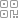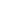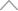6 5 1 6 1 8 8

# 举个栗子！Tableau 技巧（244）：用和弦图（Chord diagram）呈现数据关系https://www.dkmeco.com/cms/course/detail/645

D▲ sheet 1

- 角色 ID ：用于识别角色的唯一 ID，同一人物 角色 ID 和关系人 ID 相同；

- 关系人 ID ：用于识别关系人的唯一 ID，同一人物 角色 ID 和关系人 ID 相同；

- 主要关系类型 ：对角色和关系人的关系的离散分类；

- 关系描述 ：对角色和关系人的关系的文字描述，缺失此字段不影响图形展示；

- 连接 ：lonely moon 表和 repetition 表内连的字段，相同即可。sheet 2 ▲

- R ：范围从 0.01~1 共计 100 个数，以及单独的 2。此字段的目的为了在 Tableau 连接两个表，将 lonely moon 的数据重复 101 遍；

- 连接 ：与 lonely moon 的连接相同，都为1，方便“内连接”。

Tips：所有的人物 ID 都需要有一行角色 ID，即使该人物 ID没有对应的关系人 ID，例如：孙光阳；虽然关系是相互的，但是在数据中，若已经有了金刚鼠->孙光阳的关系，即可不必补充孙光阳->金刚鼠的关系。

>>>>>>>>>>>>

➤ 计算字段：各角色最小关系id ，键入函数：

{ FIXED [角色id]:MIN([关系人id])}，该字段用于限制只出现一个头像点 。➤ 计算字段：总人数 ，键入函数：

{fixed:COUNTD([角色id])}，该字段用于三角函数使用。➤ 计算字段：标签 ，键入函数：

IF [R] = 2 then [角色] END，该字段用于显示头像的名称。➤ 计算字段：突出点 ，键入函数：

if ([突出关系人]=[角色] or [突出角色]=[角色]) and [R]=2

and [关系人id]=[各角色最小关系id] then '可突出'

ELSEIF [突出角色]=[角色] and [R]!=2 and [突出关系人]=[关系人] then '可突出'

else '不可突出' end➤ 计算字段：关系人.x ，键入函数：

COS([关系人id]/[总人数] * PI() * 2)  //范围cos(2Π/n ~2Π) [-1，1）➤ 计算字段：关系人.y ，键入函数：

sin([关系人id]/[总人数] * PI() * 2)  //范围sin(2Π/n ~2Π) [-1，1）➤ 计算字段：角色.x ，键入函数：

COS([角色id]/[总人数] * PI() * 2)    //范围cos(2Π/n ~2Π) [-1，1）➤ 计算字段：角色.y ，键入函数：

sin([角色id]/[总人数] * PI() * 2)     //范围sin(2Π/n ~2Π) [-1，1）➤ 计算字段：余弦.x ，键入函数：

COS((ACOS([角色.x])+ACOS([关系人.x]))/2)➤ 计算字段：正弦.y ，键入函数：

SIN((ASIN([关系人.y])+ASIN([角色.y]))/2)➤ 计算字段：距离 ，键入函数：

SQRT(([角色.x] - [关系人.x])^2 + ([角色.y] - [关系人.y])^2)➤ 计算字段：x ，键入函数：

IF [R] >= 0 AND [R] <= 1

THEN (( (1 - [R])^2 ) * [角色.x]) + (2 * (1 - [R]) * [R] *  ( ((2 - [距离])/ 2) *[余弦.x] )) + ([R]^2 * [关系人.x]) END➤ 计算字段：y ，键入函数：

IF [R] >= 0 AND [R] <= 1

THEN (( (1 - [R])^2 ) * [角色.y]) + (2 * (1 - [R]) * [R] *   ( ((2 - [距离])/ 2) * [正弦.y]  ) ) + ([R]^2 * [关系人.y])

ELSEIF [R] = 2 and [关系人id]=[各角色最小关系id]

THEN [distance] * [角色.y] END➤ 计算字段：x.shape ，键入函数：

IF [R] = 2  and [关系人id]=[各角色最小关系id]

THEN [distance] * [角色.x] END>>>>>>>>➤ 参数：传递需要突出的关系人， 如下图设置。➤ 参数：传递需要突出的角色 ，如下图设置。➤ 突出显示动作：突出显示1， 如下图设置。## 新知精选

### 优选课程 新知学院

•扫码下载商业新知APP~

•回到顶部